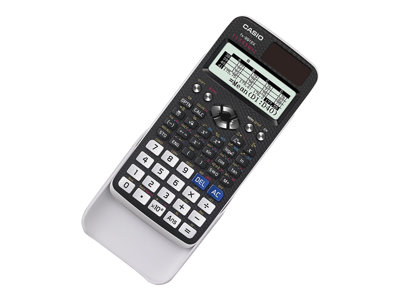Roll over main image to zoom in. Click to open expanded view.

# Casio ClassWiz FX-991EX - Scientific calculator - battery

Price:
\$3399

## 079767032593

The ClassWiz features a high-resolution display - making it easier to view numerical formulas and symbols. The ClassWiz makes it possible to display on the screen twice the number of characters in normal size and six times the number in small size compared with previous models. A high-performance processor and twice the memory of previous models ensure speedy operation and superior computational power.

Casio ClassWiz FX-991EX - Scientific calculator - battery
General
Product Type:
• Scientific calculator
Power Source:
• Battery
Features:
• 9 memory registers, QR code reader
Functions
Basic:
• Addition, subtraction, multiplication, division, undo
Scientific:
• Trigonometry, coordinate conversion, permutation, combination, fraction, complex numbers, standard deviation, matrix, power regression, differential, spreadsheet, vectors, prime factorization, regression analysis, random integer
Graphing:
• Solve functions
Display
Type:
• LCD
• High-resolution LCD drives further evolution
Higher resolution increases the amount of information that can be displayed and improves usability. In addition, ClassWiz is equipped with a basic spreadsheet function for creating spreadsheets with up to 5 columns and 45 rows (maximum of 170 data items).
• Intuitive icon display
The use of icons on the menu screen improves viewability. Selection of the desired function is quick and easy.
• Interactive format
Interactive menu display realizes more intuitive operation.
• Natural Textbook display
You can input and display fractions, powers, logarithms, roots, and other mathematical formulas and symbols just as they appear in textbooks.
• Special surface processing
A special surface process applied to the body results in an elegant texture and design.
• Key functions arranged by color
The functions assigned to keys are color-coded to facilitate key operation.
• Easy-to-understand key notation
The key notation, like the display format, uses signs and symbols like those found in mathematics textbooks. This natural interface makes input fast and easy.
• Thin rounded body
The thin, smoothly rounded body fits the hand perfectly.
• High computational competence for performing even advanced mathematics
ClassWiz contains calculation functions that support even advanced mathematical operations, including spreadsheet calculations, 4 X 4 matrix calculations, calculation of simultaneous equations with four unknowns and quartic equations, and advanced statistical distribution calculations.

S15779730 Casio FX991EX 079767032593 Casio ClassWiz FX-991EX new
33.99 USD InStock
©2000-2023 Howard Technology Solutions, a division of Howard Industries, Inc.